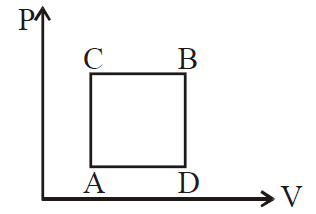# Q. A gas can be taken from A to B via two different processes ACB and ADB. When path ACB is used 60 J of heat flows into the system and 30 J of work is done by the system. If path ADB is used work done by the system is 10 J. The heat Flow into the system in path ADB is :JEE Main JEE Main 2019

Solution:

## $\Delta Q_{ACB} = \Delta W_{ACB} + \Delta U_{ACB}$ $\Rightarrow \; 60 J = 30 J + \Delta U_{ACB}$ $\Rightarrow \; \Delta U_{ACB} = 30 \;J$ $\Rightarrow \; \Delta U_{ADB} = \Delta U_{ACB } = 30 \; J$ $\Delta Q_{ACD} = \Delta U_{ACB} + \Delta W_{ADB}$ = 10 J + 30 J = 40 JYou must select option to get answer and solution

## 1. The potential to which a conductor is raised, depends on ........

KCET 2005 Electrostatic Potential And Capacitance

## 2. The refractive index of a particular material is 1.67 for blue light, 1.65 for yellow light and 1.63 for red light. The dispersive power of the material is .........

KCET 2004 Electrostatic Potential And Capacitance

## 3. In a permanent magnet at room temperature

KCET 2019 Moving Charges And Magnetism

## 4. An antenna uses electromagnetic waves of frequency 5 MHz. For proper working, the size of the antenna should be

KCET 2019 Communication Systems

## 5. In the following circuit, what are P and Q?

KCET 2019 Semiconductor Electronics: Materials Devices And Simple Circuits

## 6. Two metal plates are separated by 2 cm. The potentials of the plates are − 10 V and + 30 V. The electric field between the two plates is

KCET 2019 Electrostatic Potential And Capacitance

KCET 2019 Nuclei

## 8. For a transistor amplifier, the voltage gain

KCET 2019 Semiconductor Electronics: Materials Devices And Simple Circuits

## 9. “Parsec” is the unit of

BHU 2007 Physical World, Units and Measurements

## 10. “Parsec” is the unit of

BHU 2007 Physical World, Units and Measurements